# AAMP - Matrix Profile

This notebook is used to implement the AAMP algorithm of the matrix profile from the following paper:

https://arxiv.org/pdf/1901.05708.pdf

It claims to be faster than the Keogh and Mueen algorithm SCRIMP++. It computes the distances with a non-normalized Euclidean distance.

In :
from IPython.display import Image

In :
Image('images/matrixprofileAAMP.PNG')

Out: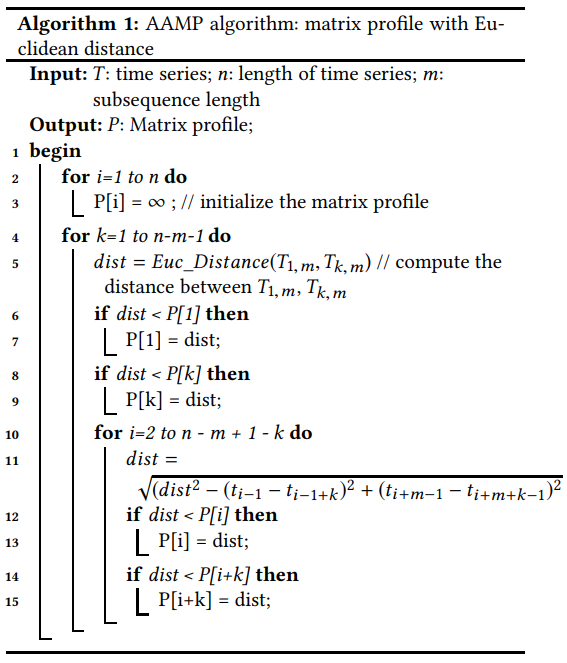In :
import numpy as np

In :
sampledata = np.loadtxt('sampledata.txt')

In :
import matplotlib.pyplot as plt

%matplotlib inline

In :
from matrixprofile.matrixProfile import stomp

In :
import numpy as np

def aamp(t, m):
"""
Computes the matrix profile using squared distance to optimize
computation.

Parameters
----------
t : np.array
Series object to compute the matrix profile.
m : int
Window size.

Returns
-------
tuple : (np.array, np.array)
The matrix profile and matrix profile index.
"""
t = np.array(t)

# initialize profile
n = len(t)
s = n - m
p = np.full(s, np.inf)
pi = np.full(s, np.inf)

# Additional optimization - Powers of 2 are better to compute than
# odd numbers.
if len(t) % 2 == 1:
t = np.insert(t, 0, 0)

for k in range(s - 1):
a = t[0:m]
b = t[k+1:m+k+1]
d = np.sum((a - b) ** 2)

if d < p:
p = d
pi = k

if d < p[k]:
p[k] = d
pi[k] = 0

for i in range(s - k - 1):
kplusi = k + i + 1
d = d - (t[i] - t[kplusi]) ** 2 + (t[m+i] - t[m+kplusi]) ** 2

if p[i] > d:
p[i] = d
pi[i] = kplusi

if p[kplusi] > d:
p[kplusi] = d
pi[kplusi] = i

p = np.sqrt(p)
return (p, pi)

In :
a = np.array([1, 2, 3])
a ** 2

Out:
array([1, 4, 9])

### Experiment 1¶

In this experiment I compare STOMP with the AAMP algorithm on a synthetic data set.

In :
t = sampledata
m = 32

In :
plt.figure(figsize=(20, 5))
plt.plot(range(len(t)), t)
plt.title('Synthetic Data')
plt.show()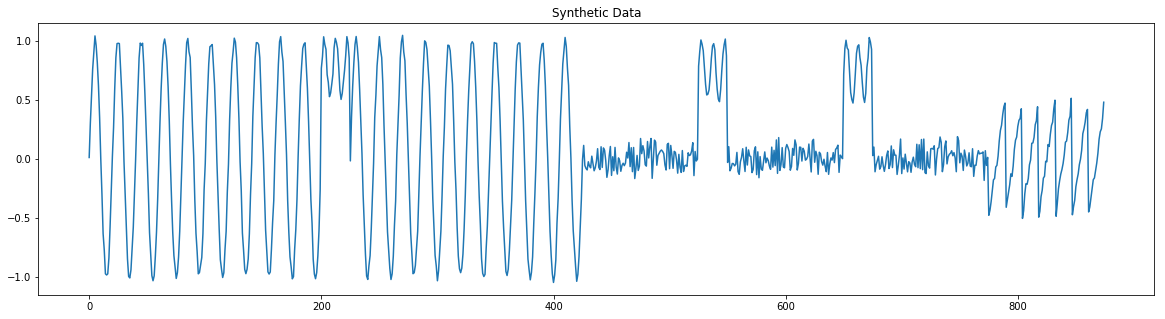In :
%%timeit
p, pi = aamp(t, m)

976 ms ± 55.3 ms per loop (mean ± std. dev. of 7 runs, 1 loop each)

In :
p, pi = aamp(t, m)

In :
%%timeit
stomp_mp, stomp_idx = stomp(np.array(t), m)

263 ms ± 34.1 ms per loop (mean ± std. dev. of 7 runs, 1 loop each)

In :
stomp_mp, stomp_idx = stomp(np.array(t), m)

In :
#Plot the signal data
fig, (ax1, ax2, ax3) = plt.subplots(3,1,sharex=True,figsize=(20,5))
ax1.plot(np.arange(len(t)), t, label="Synthetic Data")
ax1.set_ylabel('Signal', size=12)

#Plot the Matrix Profile
ax2.set_ylabel('AAMP', size=12)

#Plot the Matrix Profile
mp_adj = np.append(stomp_mp, np.zeros(m-1)+np.nan)
ax3.set_ylabel('STOMP', size=12)
ax3.set_xlabel('Sample', size=12)

plt.tight_layout()
plt.show()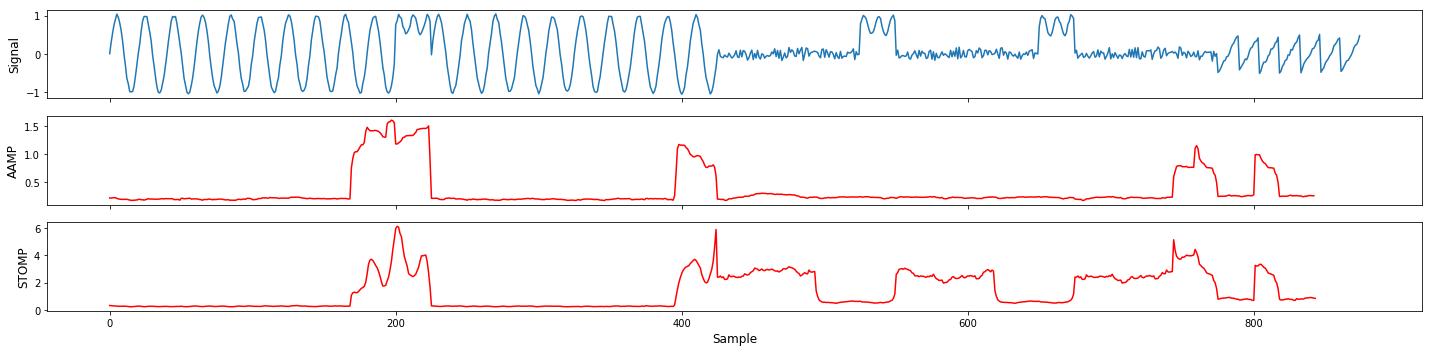In the first expirment, you see that STOMP is significantly faster when compared in Python. The research paper claims that the window size (m) and series length (n) are major factors when comparing the algorithms. Note that in the paper they compare SCRIMP++. However, at this time I do not have a Python implementation of SCRIMP++. Also, STOMP is faster than SCRIMP++ according to Keogh's paper, Matrix Proﬁle XI: SCRIMP++: Time Series Motif Discovery at Interactive Speed.

The distance profiles of both the AAMP and STOMP algorithm differ. This is due to the differences in calculation. The AAMP algorith does not Z-Normalize the data.

### Experiment 2¶

In this experiment I generate a uniform distribution and follow what the research claims; m an n are major factors in time complexity.

In :
t = np.random.uniform(size=2**10)
m = 2 ** 5

In :
plt.figure(figsize=(20, 5))
plt.plot(range(len(t)), t)
plt.title('Synthetic Data')
plt.show()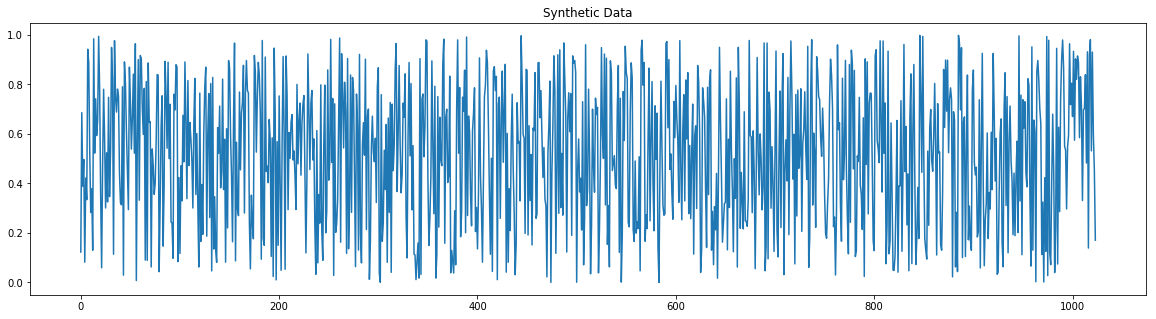In :
%%timeit
p, pi = aamp(t, m)

1.3 s ± 36.2 ms per loop (mean ± std. dev. of 7 runs, 1 loop each)

In :
%%timeit
stomp_mp, stomp_idx = stomp(np.array(t), m)

285 ms ± 9.4 ms per loop (mean ± std. dev. of 7 runs, 1 loop each)


In this experiment we see that STOMP is still faster.

### Experiment 3 - Matlab runtimes of AAMP vs SCRIMP++¶

I reached out to the author of the paper to identify any issues with their claims. They provided me with the Matlab code used in their experiments. This experiment compares Matlab implementations to one another.

In :
Image('images/matlab_scrimp_aamp.PNG')

Out: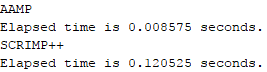The AAMP algorithm performs significantly faster than the SCRIMP++ algorithm within Matlab. I'm not too familiar with Matlab as a whole, but it seems that there is some sort of optimization that lacks within the Python implementation.

Contents © 2020 Tyler Marrs